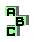BEVAN POINT

 r76 excenters r74 excentral triangle, circumcenter d point X(84) r132 Nagel point, De Longchamps point r193 Schröder point, Weill point,Bevan-Schröder point,poristic triangles, point X(65), point X(55), point X(56) r280 orthocenter, Spieker point, Mittenpunkt r316 r356 point X(63) r357 perspective, extouch triangle, excentral triangle r405 excircles, radical center r434 Bevan-Schröder point, extouch triangle r531 r571 Spieker point, incenter, anticomplement, orthopole, circumconic r572 orthocenter, Spieker point, Clawson point, Mittenpunkt r595 excenters, circumcenter, De Longchamps point, point X(84), isogonal points, desmic structure r641 extouch triangle, circumcenter, point X(84) r652 excenters, Feuerbach point, point X(100) d Longuet-Higgins point r724 point X(165), incenter, circumcenter, harmonic points r725 point X(165), centroid, orthocenter, De Longchamps point r739 incenter, excentral triangle, orthocenter, circumcenter, Mittenpunkt, Prasolov point,point X(64),point X(84) r792 orthocenter, incenter, complement r1057 incenter, excenters, circumcenter, ninecenter r1638 circumcevian triangle, perspective, incenter, circumcenter r1922 circumcenter, ninecenter, medial triangle, excentral triangle